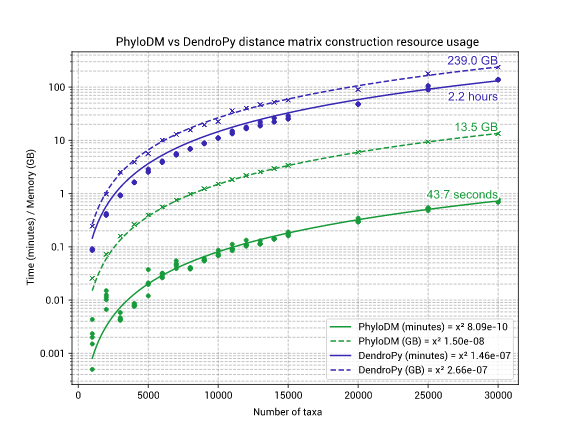## bin+lib phylodm

Efficient calculation of phylogenetic distance matrices

### 8 stable releases

 3.0.0 Jun 17, 2023 Jan 9, 2023 Oct 4, 2022 Jul 28, 2022 May 3, 2022

#304 in Math

135KB
1K SLoC

# 🌲 PhyloDM

PhyloDM is a high-performance library that converts a phylogenetic tree into a pairwise distance matrix.

For a tree with 30,000 taxa, PhyloDM will use:

• ~14GB of memory (94% less than DendroPy)
• ~1 minute of CPU time (183x faster than DendroPy).

PhyloDM is written in Rust and is exposed to Python via the Python PyO3 API. This means it can be used in either Python or Rust, however, the documentation below is written for use in Python. For Rust documentation, see Crates.io.

## ⚙ Installation

Requires Python 3.7+

### PyPI

Pre-compiled binaries are packaged for most 64-bit Unix platforms. If you are installing on a different platform then you will need to have Rust installed to compile the binaries.

``````python -m pip install phylodm
``````

### Conda

``````conda install -c b bioconda phylodm
``````

## 🐍 Quick-start

A pairwise distance matrix can be created from either a Newick file, or DendroPy tree.

``````from phylodm import PhyloDM

# PREPARATION: Create a test tree
with open('/tmp/newick.tree', 'w') as fh:
fh.write('(A:4,(B:3,C:4):1);')

# 1a. From a Newick file

# 1b. From a DendroPy tree
import dendropy
tree = dendropy.Tree.get_from_path('/tmp/newick.tree', schema='newick')

# 2. Calculate the PDM
dm = pdm.dm(norm=False)
labels = pdm.taxa()

"""
/------------------------ A
+
|          /------------------ B
\------+
\------------------------- C

labels = ('A', 'B', 'C')
dm = [[0. 8. 9.]
[8. 0. 7.]
[9. 7. 0.]]
"""
``````

### Accessing data

The `dm` method generates a symmetrical NumPy matrix and returns a tuple of keys in the matrix row/column order.

``````# Calculate the PDM
dm = pdm.dm(norm=False)
labels = pdm.taxa()

"""
/------------------------ A
+
|          /------------------ B
\------+
\------------------------- C

labels = ('A', 'B', 'C')
dm = [[0. 8. 9.]
[8. 0. 7.]
[9. 7. 0.]]
"""

# e.g. The following commands (equivalent) get the distance between A and B
dm[0, 1]  # 8
dm[labels.index('A'), labels.index('B')]  # 8
``````

### Normalisation

If the `norm` argument of `dm` is set to `True`, then the data will be normalised by the sum of all edges in the tree.

## ⏱ Performance

Tests were executed using `scripts/performance/Snakefile` on an Intel(R) Xeon(R) CPU E5-2650 v3 @ 2.30GHz.

For large numbers of taxa it is beneficial to use PhyloDM, however, if you have a small number of taxa in the tree it is beneficial to use DendroPy for the great features it provides.~8–15MB
~180K SLoC